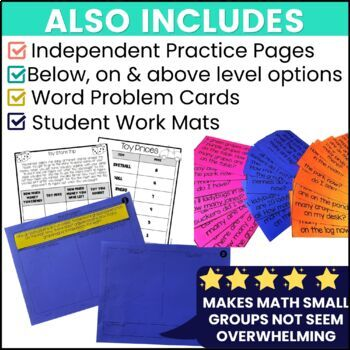# 1st grade Word Problem Activities - Subtraction Word Problem Games1st
Subjects
Standards
Resource Type
Formats Included
• PDF
Pages
389 pages
##### Also included in
1. Set your year up for success with this full-year 1st grade math curriculum! These ready to use math resources come with 14 units that provide differentiated small group math instruction for 1st graders.  Having engaging and fun 1st grade math centers will allow your students to learn and thrive in y
\$92.40
\$131.00
Save \$38.60

### Description

1st Grade Addition and subtraction word problems or contextual problems small group math will help your students to develop the skills they need to master math word problems addition and subtraction within 20. These small group math activities, challenges, and games are differentiated to teach your students exactly what they need to know in order to master the 1st grade common core math standards 1.OA.A.1, 1.OA.C.5, 1.OA.C.6 in no time!

Why are these lessons for 1st Grade Addition and Subtraction Word Problems

different from others you have seen?

First, the word problem lessons are differentiated but not just by saying to assist more or less with each level group. There are differentiated contextual problem work mats, equation cards, independent practice pages and more for 3 levels of groups. Second, these math word problems addition and subtraction within 20 are meant to be ink friendly and very low prep. Almost every page in these lessons can be printed on color paper or even just plain white paper to save on ink (even the games)!

The prep for these 1st grade small group math activities is teacher friendly! Prep for these math word problems addition and subtraction within 20 consists of printing the lesson plans, work mats front to back for each group, and games. Use dry erase pocket sleeves with the work mats and laminate the games and then save these contextual problem materials to use year after year! The only thing that will need to be reprinted each year are independent practice pages.

What is included in this 1st Grade Addition and subtraction word problems packet

✏️10 lesson plans

✏️Differentiated student work mats

✏️Differentiated independent practice pages

✏️Games with each lesson

✏️Challenges at the end of each lesson

✏️Differentiated word problems to use in each lesson

Small Group Math Activities and Lessons Include

❤️Add To Change Unknown Day 1

❤️Add To Change Unknown Day 2

❤️Take From Change Unknown Day 1

❤️Take From Change Unknown Day 2

❤️Put Together/Take Apart Addend Unknown Day 1

❤️Put Together/Take Apart Addend Unknown Day 2

❤️Compare/Difference Unknown How Many More

❤️Compare/Difference Unknown How Many Fewer

❤️Compare Bigger Unknown Version With More

❤️Compare Bigger Unknown Version With Fewer

You may also like:

Unit 1: Addition and Subtraction Strategies (1.OA.A.1, 1.OA.C.5, 1.OA. A. 6)

Unit 2: The Equal Sign & Balancing Equations (1.OA.B.3, 1.OA.C.5, 1.OA.D.7, 1.OA.D.8)

Unit 3 Missing Addends & Related Facts (1.OA.B.4, 1.OA.C.5, 1.OA.C.6)

Unit 4 Measurement/Ordering and Comparing Lengths (1.MD.A.1, 1.MD.A.2)

Unit 5 Word Problems(1.OA.A.1, 1.OA.C.5, 1.OA.C.6, 1.OA.D.8)

Unit 6 Adding 3 Numbers (1.OA.A.2, 1.OA.B.3, 1.OA.C.6)

Unit 8 - Graphing (1.OA.A.1, 1.OA.C.6, 1.MD.C.4)

Unit 9 Using Place Value to Read, Write, Represent, and Compare Numbers (1.NBT.B.2., 1.NBT.B.3)

Unit 10 Adding Multiples of Ten (1.NBT.A.1, 1.NBT.C.4)

Shapes and their Attributes (1.G.A.1, 1.G.A.2)

Fractions/Partitioning Shapes (1.G.A.3)

≒≒≒≒≒≒≒≒≒≒≒≒≒≒≒≒≒≒≒≒≒≒≒≒≒≒≒≒≒≒≒≒≒≒≒≒≒≒≒≒≒≒≒≒≒≒≒≒

Don't forget to leave feedback. You will receive TPT credits that can be used on future purchases!

Want FREE Resources?

FREE Differentiated Math Word Problem Activity

FREE Retelling Lesson Plan

FREE Interactive Read Aloud 5 Day Lesson Plan

FREE Main Idea Printables

Connect with me:

Pinterest

Instagram

FREE Main Idea Printables

Connect with me:

Pinterest

Instagram

Total Pages
389 pages
N/A
Teaching Duration
N/A
Report this Resource to TpT
Reported resources will be reviewed by our team. Report this resource to let us know if this resource violates TpT’s content guidelines.

### Standards

to see state-specific standards (only available in the US).
Use addition and subtraction within 20 to solve word problems involving situations of adding to, taking from, putting together, taking apart, and comparing, with unknowns in all positions, e.g., by using objects, drawings, and equations with a symbol for the unknown number to represent the problem.
Relate counting to addition and subtraction (e.g., by counting on 2 to add 2).
Add and subtract within 20, demonstrating fluency for addition and subtraction within 10. Use strategies such as counting on; making ten (e.g., 8 + 6 = 8 + 2 + 4 = 10 + 4 = 14); decomposing a number leading to a ten (e.g., 13 - 4 = 13 - 3 - 1 = 10 - 1 = 9); using the relationship between addition and subtraction (e.g., knowing that 8 + 4 = 12, one knows 12 - 8 = 4); and creating equivalent but easier or known sums (e.g., adding 6 + 7 by creating the known equivalent 6 + 6 + 1 = 12 + 1 = 13).